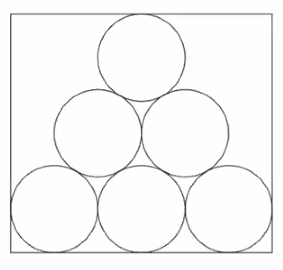# Area of multiple circles inside a rectangle

• MHB
Help seeker
Figure shows six identical circles inside a rectangle.The radius of each circle is 24 cm. The radius of the circles is the greatest possible radius so that the circles fit inside the rectangle. The six circles form the pattern shown in Figure so that
• each circle touches at least two other circles
• the circle in the top row of the pattern and the circles in the bottom row of the pattern touch at least one side of the rectangle
• the centres of the circles all lie on the perimeter of a single triangle.

Find the total area of the $six$ $circles$ $as$ $a$ $percentage$ $of$ $the$ $area$ $of$ $the$ $rectangle$.

Gold Member
MHB
Height of triangle = √(96²-48²) ≈ 83.1384387633 cm
Area of rectangle ≈ 144×(83.1384387633+48) ≈ 18883.9351819 cm²
Area of 6 circles ≈ 10857.34422 cm²
Area of 6 circles as a percentage of area of rectangle ≈ 57.49513603 %

jonah1
Height of triangle = √(96²-48²) ≈ 83.1384387633 cm
Area of rectangle ≈ 144×(83.1384387633+48) ≈ 18883.9351819 cm²
Area of 6 circles ≈ 10857.3442$$\displaystyle {\color{red}2}$$ cm²
Area of 6 circles as a percentage of area of rectangle ≈ 57.49513$$\displaystyle {\color{red}603}$$ %
Minor oversight or calculator algorithm difference.
Those might have been
Area of 6 circles ≈ 10857.3442108
and
Area of 6 circles as a percentage of area of rectangle ≈ 57.4951359778

Help seeker
Tnx
Solved